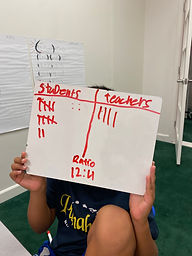## Ms. Kylie

### Target 1​

###### Lesson Type:

New

Data Probability

:

Ratio

Solve problems involving ratios.

###### 1:

Use ratio vocabulary to describe the relationships between two quantities.

###### 2:

Understand that a ratio expresses the comparison between two quantities.

6th

###### Vocabulary:

Ratio, Comparison

Activities:

-Students learned a ratio is a comparison between quantities. Students found the ratio of students to teachers in the learning center. They also practiced finding the ratio of two colored cubes.

-Students were given task cards with different scenarios of describing relationships between two quantities. They wrote sentences describing the ratio relationship and wrote the ratio using a colon.### Home Exploration

###### Guiding Questions:## Absent Students:

### Target 2

:

###### 1:

Use the corresponding sides of similar triangles to determine the unknown length of a side.

###### 2:

Find the ratio of corresponding sides and use that ratio to find unknown lengths.

6th

###### Vocabulary:

Ratio, Corresponding Sides, Congruent

Activities:

-Students practiced finding the ratio for the corresponding sides of two triangles. They used the ratio to find the unknown length of a triangle.

-Students practiced finding the missing angles of a triangle by adding the two given angles and subtracting it by 180 degrees. Students understood similar triangles have corresponding angles that are congruent.### Home Exploration

###### Guiding Questions:### Target 3

:

###### 1:

Identify the correct arithmetic process based on the information presented in word problems.

###### 2:

Find the key words in word problems that indicate the correct arithmetic process to use.

###### 3:

Find and use the needed information in a word problem to solve.

5th

###### Vocabulary:

CUBES, Operation Words

Activities:

-Students used the strategy CUBES to solve multi-step word problems.

-Students were given multi-step word problems to solve using CUBES to identify the correct operations and information needed.### Home Exploration